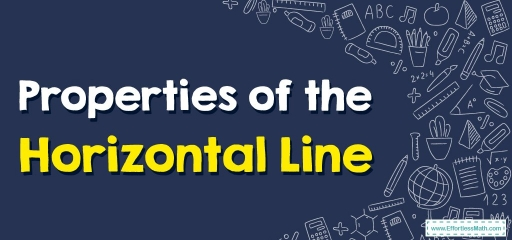# Properties of the Horizontal Line

A horizontal line is a straight line that is drawn from left to right or right to left and is parallel to the $$x$$-axis in the coordinate plane. Here you get familiarized with the properties of the horizontal line, its equation, and the slope of a horizontal line.A straight line that has an intercept only on the $$y$$-axis, not on the $$x$$-axis is called a horizontal line. The base that we draw for flat shapes is a horizontal line.

## A step-by-step guide to properties of the horizontal line

Horizontal lines are also known as sleeping lines. In coordinate geometry, horizontal lines are lines that are parallel to the $$x$$-axis. In geometry, we can find horizontal line segments in various shapes such as quadrilaterals, $$3$$D, and so on.

### The slope of the horizontal line

The slope of a horizontal line is zero. While calculating $$slope=\frac{rise}{run}$$, we see that there is no increases in the $$y$$ coordinates because they are the same across the horizontal line. So there are no changes in the $$y$$ coordinate and finally, the slope of the horizontal line is $$0$$.

### Horizontal line equation

According to the previous image, we see that the $$y$$ coordinates of all points on a horizontal line are the same. Thus, the equation of a horizontal line through any point $$(a,b)$$ is of the form: $$y = b$$, where $$b$$ is constant.

Here $$x$$ is absent. It means that the $$x$$-coordinate can be anything whereas the $$y$$-coordinate of all the points on the line must be $$b$$ only. The $$y$$-intercept of the horizontal line is $$(0, b)$$.

### Horizontal line test

Horizontal line testing is used to determine whether a function is a one-to-one function. According to the horizontal line test, if there is a horizontal line that passes through more than one point of the graph (function), it is NOT a one-to-one function. In other words, in a one-to-one function, there is only one unique $$x$$ value for each $$y$$ value.

### Drawing horizontal lines

• To draw a horizontal line, we need to know the dimensions of the point (i.e.,$$x$$ and $$y$$ value).
• Now, we can locate the point in the $$xy$$ plane.
• Taking the point $$(x,y)$$ as the reference point, we need to draw a line parallel to the $$x$$-axis.
• Thus, a horizontal line is drawn.

### Properties of the Horizontal Line –Example 1:

Draw the horizontal line $$y = -3$$.

## Exercises for Properties of the Horizontal Line

1. Find the equation of the horizontal line passing through the point $$(3,8)$$.
2. Determine the horizontal line equation, whose $$y$$-intercept is $$(0, 2)$$.
3. If a horizontal line passes through the point $$(1,-5)$$, what is the equation of the line?
1. $$\color{blue}{y=8}$$
2. $$\color{blue}{y=2}$$
3. $$\color{blue}{y=-5}$$

### What people say about "Properties of the Horizontal Line - Effortless Math: We Help Students Learn to LOVE Mathematics"?

No one replied yet.

X
30% OFF

Limited time only!

Save Over 30%

SAVE $5 It was$16.99 now it is \$11.99